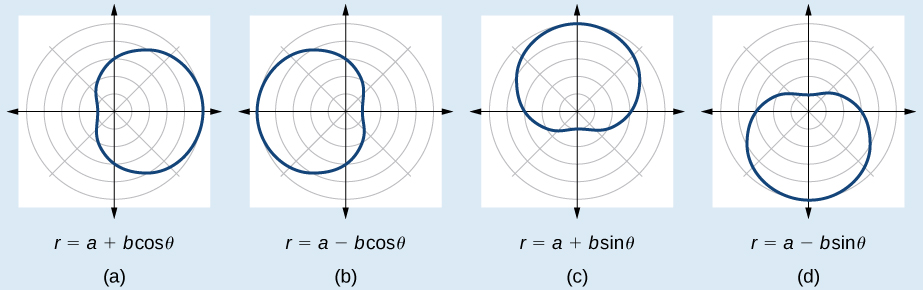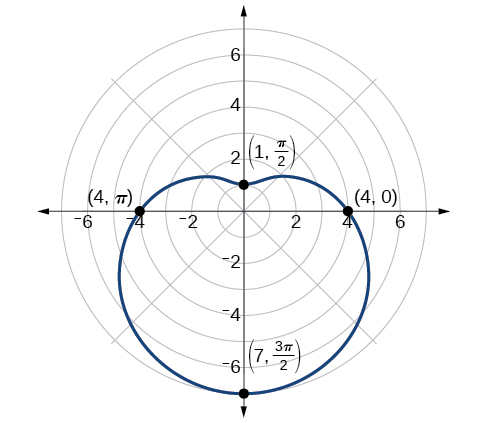# 10.4 Polar coordinates: graphs  (Page 4/16)

 Page 4 / 16

## Formulas for a cardioid

The formulas that produce the graphs of a cardioid    are given by $\text{\hspace{0.17em}}r=a±b\mathrm{cos}\text{\hspace{0.17em}}\theta \text{\hspace{0.17em}}$ and $\text{\hspace{0.17em}}r=a±b\mathrm{sin}\text{\hspace{0.17em}}\theta \text{\hspace{0.17em}}$ where $\text{\hspace{0.17em}}a>0,\text{\hspace{0.17em}}\text{\hspace{0.17em}}b>0,\text{\hspace{0.17em}}$ and $\text{\hspace{0.17em}}\frac{a}{b}=1.\text{\hspace{0.17em}}$ The cardioid graph passes through the pole, as we can see in [link] .

Given the polar equation of a cardioid, sketch its graph.

1. Check equation for the three types of symmetry.
2. Find the zeros. Set $\text{\hspace{0.17em}}r=0.$
3. Find the maximum value of the equation according to the maximum value of the trigonometric expression.
4. Make a table of values for $\text{\hspace{0.17em}}r\text{\hspace{0.17em}}$ and $\text{\hspace{0.17em}}\theta .$
5. Plot the points and sketch the graph.

## Sketching the graph of a cardioid

Sketch the graph of $\text{\hspace{0.17em}}r=2+2\mathrm{cos}\text{\hspace{0.17em}}\theta .$

First, testing the equation for symmetry, we find that the graph of this equation will be symmetric about the polar axis. Next, we find the zeros and maximums. Setting $\text{\hspace{0.17em}}r=0,\text{\hspace{0.17em}}$ we have $\text{\hspace{0.17em}}\theta =\pi +2k\pi .\text{\hspace{0.17em}}$ The zero of the equation is located at $\text{\hspace{0.17em}}\left(0,\pi \right).\text{\hspace{0.17em}}$ The graph passes through this point.

The maximum value of $\text{\hspace{0.17em}}r=2+2\mathrm{cos}\text{\hspace{0.17em}}\theta \text{\hspace{0.17em}}$ occurs when $\text{\hspace{0.17em}}\mathrm{cos}\text{\hspace{0.17em}}\theta \text{\hspace{0.17em}}$ is a maximum, which is when $\text{\hspace{0.17em}}\mathrm{cos}\text{\hspace{0.17em}}\theta =1\text{\hspace{0.17em}}$ or when $\text{\hspace{0.17em}}\theta =0.\text{\hspace{0.17em}}$ Substitute $\text{\hspace{0.17em}}\theta =0\text{\hspace{0.17em}}$ into the equation, and solve for $\text{\hspace{0.17em}}r.\text{\hspace{0.17em}}$

$\begin{array}{l}\begin{array}{l}\\ r=2+2\mathrm{cos}\left(0\right)\end{array}\hfill \\ r=2+2\left(1\right)=4\hfill \end{array}$

The point $\text{\hspace{0.17em}}\left(4,0\right)\text{\hspace{0.17em}}$ is the maximum value on the graph.

We found that the polar equation is symmetric with respect to the polar axis, but as it extends to all four quadrants, we need to plot values over the interval $\text{\hspace{0.17em}}\left[0,\text{\hspace{0.17em}}\pi \right].\text{\hspace{0.17em}}$ The upper portion of the graph is then reflected over the polar axis. Next, we make a table of values, as in [link] , and then we plot the points and draw the graph. See [link] .

 $\theta$ $0$ $\frac{\pi }{4}$ $\frac{\pi }{2}$ $\frac{2\pi }{3}$ $\pi$ $r$ 4 3.41 2 1 0

## Investigating limaçons

The word limaçon is Old French for “snail,” a name that describes the shape of the graph. As mentioned earlier, the cardioid is a member of the limaçon family, and we can see the similarities in the graphs. The other images in this category include the one-loop limaçon and the two-loop (or inner-loop) limaçon. One-loop limaçons are sometimes referred to as dimpled limaçons when $\text{\hspace{0.17em}}1<\frac{a}{b}<2\text{\hspace{0.17em}}$ and convex limaçons when $\text{\hspace{0.17em}}\frac{a}{b}\ge 2.\text{\hspace{0.17em}}$

## Formulas for one-loop limaçons

The formulas that produce the graph of a dimpled one-loop limaçon are given by $\text{\hspace{0.17em}}r=a±b\mathrm{cos}\text{\hspace{0.17em}}\theta \text{\hspace{0.17em}}$ and $\text{\hspace{0.17em}}r=a±b\mathrm{sin}\text{\hspace{0.17em}}\theta \text{\hspace{0.17em}}$ where All four graphs are shown in [link] .Dimpled limaçons

Given a polar equation for a one-loop limaçon, sketch the graph.

1. Test the equation for symmetry. Remember that failing a symmetry test does not mean that the shape will not exhibit symmetry. Often the symmetry may reveal itself when the points are plotted.
2. Find the zeros.
3. Find the maximum values according to the trigonometric expression.
4. Make a table.
5. Plot the points and sketch the graph.

## Sketching the graph of a one-loop limaçon

Graph the equation $\text{\hspace{0.17em}}r=4-3\mathrm{sin}\text{\hspace{0.17em}}\theta .$

First, testing the equation for symmetry, we find that it fails all three symmetry tests, meaning that the graph may or may not exhibit symmetry, so we cannot use the symmetry to help us graph it. However, this equation has a graph that clearly displays symmetry with respect to the line $\text{\hspace{0.17em}}\theta =\frac{\pi }{2},\text{\hspace{0.17em}}$ yet it fails all the three symmetry tests. A graphing calculator will immediately illustrate the graph’s reflective quality.

Next, we find the zeros and maximum, and plot the reflecting points to verify any symmetry. Setting $\text{\hspace{0.17em}}r=0\text{\hspace{0.17em}}$ results in $\text{\hspace{0.17em}}\theta \text{\hspace{0.17em}}$ being undefined. What does this mean? How could $\text{\hspace{0.17em}}\theta \text{\hspace{0.17em}}$ be undefined? The angle $\text{\hspace{0.17em}}\theta \text{\hspace{0.17em}}$ is undefined for any value of $\text{\hspace{0.17em}}\mathrm{sin}\text{\hspace{0.17em}}\theta >1.\text{\hspace{0.17em}}$ Therefore, $\text{\hspace{0.17em}}\theta \text{\hspace{0.17em}}$ is undefined because there is no value of $\text{\hspace{0.17em}}\theta \text{\hspace{0.17em}}$ for which $\text{\hspace{0.17em}}\mathrm{sin}\text{\hspace{0.17em}}\theta >1.\text{\hspace{0.17em}}$ Consequently, the graph does not pass through the pole. Perhaps the graph does cross the polar axis, but not at the pole. We can investigate other intercepts by calculating $r$ when $\text{\hspace{0.17em}}\theta =0.\text{\hspace{0.17em}}$

$\begin{array}{l}r\left(0\right)=4-3\mathrm{sin}\left(0\right)\hfill \\ \text{\hspace{0.17em}}\text{\hspace{0.17em}}\text{\hspace{0.17em}}\text{\hspace{0.17em}}\text{\hspace{0.17em}}\text{\hspace{0.17em}}\text{\hspace{0.17em}}r=4-3\cdot 0=4\hfill \end{array}$

So, there is at least one polar axis intercept at $\text{\hspace{0.17em}}\left(4,0\right).$

Next, as the maximum value of the sine function is 1 when $\text{\hspace{0.17em}}\theta =\frac{\pi }{2},\text{\hspace{0.17em}}$ we will substitute $\text{\hspace{0.17em}}\theta =\frac{\pi }{2}\text{\hspace{0.17em}}$ into the equation and solve for $\text{\hspace{0.17em}}r.\text{\hspace{0.17em}}$ Thus, $\text{\hspace{0.17em}}r=1.$

Make a table of the coordinates similar to [link] .

 $\theta$ $0$ $\frac{\pi }{6}$ $\frac{\pi }{3}$ $\frac{\pi }{2}$ $\frac{2\pi }{3}$ $\frac{5\pi }{6}$ $\pi$ $\frac{7\pi }{6}$ $\frac{4\pi }{3}$ $\frac{3\pi }{2}$ $\frac{5\pi }{3}$ $\frac{11\pi }{6}$ $2\pi$ $r$ 4 2.5 1.4 1 1.4 2.5 4 5.5 6.6 7 6.6 5.5 4

The graph is shown in [link] .One-loop limaçon

#### Questions & Answers

A laser rangefinder is locked on a comet approaching Earth. The distance g(x), in kilometers, of the comet after x days, for x in the interval 0 to 30 days, is given by g(x)=250,000csc(π30x). Graph g(x) on the interval [0, 35]. Evaluate g(5)  and interpret the information. What is the minimum distance between the comet and Earth? When does this occur? To which constant in the equation does this correspond? Find and discuss the meaning of any vertical asymptotes.
Kaitlyn Reply
The sequence is {1,-1,1-1.....} has
amit Reply
circular region of radious
Kainat Reply
how can we solve this problem
Joel Reply
Sin(A+B) = sinBcosA+cosBsinA
Eseka Reply
Prove it
Eseka
Please prove it
Eseka
hi
Joel
June needs 45 gallons of punch. 2 different coolers. Bigger cooler is 5 times as large as smaller cooler. How many gallons in each cooler?
Arleathia Reply
7.5 and 37.5
Nando
find the sum of 28th term of the AP 3+10+17+---------
Prince Reply
I think you should say "28 terms" instead of "28th term"
Vedant
the 28th term is 175
Nando
192
Kenneth
if sequence sn is a such that sn>0 for all n and lim sn=0than prove that lim (s1 s2............ sn) ke hole power n =n
SANDESH Reply
write down the polynomial function with root 1/3,2,-3 with solution
Gift Reply
if A and B are subspaces of V prove that (A+B)/B=A/(A-B)
Pream Reply
write down the value of each of the following in surd form a)cos(-65°) b)sin(-180°)c)tan(225°)d)tan(135°)
Oroke Reply
Prove that (sinA/1-cosA - 1-cosA/sinA) (cosA/1-sinA - 1-sinA/cosA) = 4
kiruba Reply
what is the answer to dividing negative index
Morosi Reply
In a triangle ABC prove that. (b+c)cosA+(c+a)cosB+(a+b)cisC=a+b+c.
Shivam Reply
give me the waec 2019 questions
Aaron Reply

### Read also:

#### Get the best Algebra and trigonometry course in your pocket!

Source:  OpenStax, Algebra and trigonometry. OpenStax CNX. Nov 14, 2016 Download for free at https://legacy.cnx.org/content/col11758/1.6
Google Play and the Google Play logo are trademarks of Google Inc.

Notification Switch

Would you like to follow the 'Algebra and trigonometry' conversation and receive update notifications?ByByByByBy